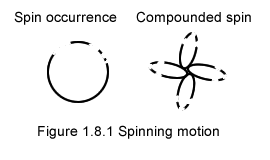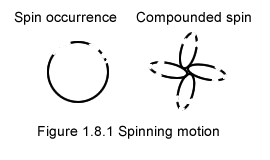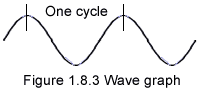Cyclic motion (§1.8)
A cyclic motion is repeating itself over and over. There are many types of cyclic motions; spinning type and pendulum type are 2 of them.
A spinning motion-occurrence inclination is curved rather than straight, and its curve remains constant just as its thrust remains constant. Figure 1.8.1 provides 2 representations of spin.A spinning motion always occurs as a combination of MOs.
Should the spin motion left of figure 1.8.1 be representing the spin of Earth, one cycle would coincide to a full revolution of Earth on itself. In gravimotion that is a motion cycle; it is neither a day nor a time; time disappeared from gravimotions interpretation of Nature.
Another cyclic motion of Earth is evidently its revolution around the Sun. In gravimotion that is another cyclic motion; years are not time but specific cyclic motions.
Whereas the inclination of Earth's spin is self contained, the inclination of Earth's rotation around the sun is controled by the sun influence.
Earth's spin is proof that motion has thrust, and is not a mere side effect of force.

A wave is another cyclic motion. Here is a picture of waves created by a passing boat.Waves are graphically represented with sine-waves, as those curves represent precisely what is shown in the left picture.The graph is not made of motion-occurrences but of a single line illustrating a real phenomenon actually made of a great number of motion-occurrences.
Axes of time and distance or amplitude would be added to this illustration in physics.
In gravimotion such a graph is referenced in terms of motion; for instance motion magnitude for the vertical axis, while the horizontal axis would be the present mo(ve)ment, just as the picture of water waves suggests.
In both physics and gravimotion, "one cycle" is defined as what happens between 2 successive identical situations, between 2 peaks for instance.
But that is where the parity stops. In gravimotion the motion occurring during the cycle is of the essence.
By contrast the motion that makes a cycle is definitely not the wave fundamental entity in physics. In case of light for instance, the wave frequency instead, is identified to energy, and becomes a fundamental aspect of the wave in physics.